## Addressing Modes in Computer Architecture-

Before you go through this article, make sure that you have gone through the previous article on Addressing Modes.

We have discussed-

• Addressing modes are the different ways of specifying the location of an operand.
• Various kinds of addressing modes and their applications.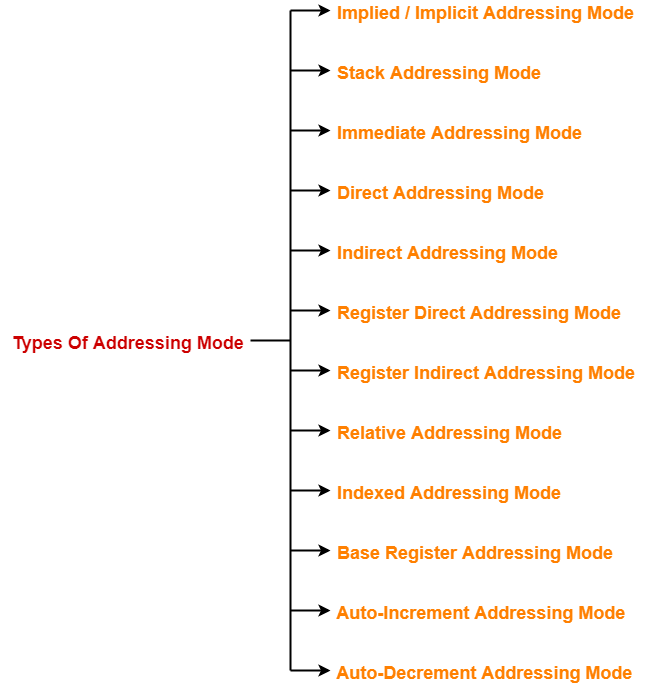## Problem-01:

The most appropriate matching for the following pairs is-

#### Column-2:

1. Loops

2. Pointers

3. Constants

1. X-3, Y-2, Z-1
2. X-1, Y-3, Z-2
3. X-2, Y-3, Z-1
4. X-3, Y-1, Z-2

## Solution-

Option (C) is correct.

## Problem-02:

1. The operand is inside the instruction
2. The address of the operand is inside the instruction
3. The register containing the address of the operand is specified inside the instruction
4. The location of the operand is implicit

## Solution-

Option (B) is correct.

## Problem-03:

Which of the following addressing modes are suitable for program relocation at run time?

1. 1 and 4
2. 1 and 2
3. 2 and 3
4. 1, 2 and 4

## Solution-

Option (C) is correct.

## Problem-04:

What is the most appropriate match for the items in the first column with the items in the second column-

#### Column-2:

1. Array implementation

2. Writing relocatable code

3. Passing array as parameter

1. X-3, Y-1, Z-2
2. X-2, Y-3, Z-1
3. X-3, Y-2, Z-1
4. X-1, Y-3, Z-2

## Solution-

Option (A) is correct.

## Problem-05:

Which of the following addressing modes permits relocation without any change whatsoever in the code?

## Solution-

Option (C) is correct.

## Problem-06:

Consider a three word machine instruction-

The first operand (destination) “A[R0]” uses indexed addressing mode with R0 as the index register. The second operand operand (source) “@B” uses indirect addressing mode. A and B are memory addresses residing at the second and the third words, respectively. The first word of the instruction specifies the opcode, the index register designation and the source and destination addressing modes. During execution of ADD instruction, the two operands are added and stored in the destination (first operand).

The number of memory cycles needed during the execution cycle of the instruction is-

1. 3
2. 4
3. 5
4. 6

## Solution-

For the first operand,

• It uses indexed addressing mode.
• Thus, one memory cycle will be needed to fetch the operand.

For the second operand,

• It uses indirect addressing mode.
• Thus, two memory cycles will be needed to fetch the operand.

After fetching the two operands,

• The operands will be added and result is stored back in the memory.
• Thus, one memory cycle will be needed to store the result.

Total number of memory cycles needed

=  1 + 2 + 1

= 4

Thus, Option (B) is correct.

## Problem-07:

Consider a hypothetical processor with an instruction of type LW R1, 20(R2), which during execution reads a 32-bit word from memory and stores it in a 32-bit register R1. The effective address of the memory location is obtained by the addition of a constant 20 and the contents of register R2. Which of the following best reflects the addressing mode implemented by this instruction for operand in memory?

## Solution-

Clearly, the instruction uses base indexed addressing mode.

Thus, Option (D) is correct.

## Problem-08:

The memory locations 1000, 1001 and 1020 have data values 18, 1 and 16 respectively before the following program is executed.

Which of the statements below is TRUE after the program is executed?

1. Memory location 1000 has value 20
2. Memory location 1020 has value 20
3. Memory location 1021 has value 20
4. Memory location 1001 has value 20

## Solution-

Before the execution of program, the memory is-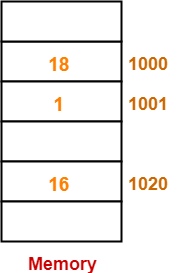Now, let us execute the program instructions one by one-

### Instruction-01: MOVI Rs, 1

• This instruction uses immediate addressing mode.
• The instruction is interpreted as Rs ← 1.
• Thus, value = 1 is moved to the register Rs.

• This instruction uses displacement addressing mode.
• The instruction is interpreted as Rd ← [1000 + [Rs]].
• Value of the operand = [1000 + [Rs]] = [1000 + 1] =  = 1.
• Thus, value = 1 is moved to the register Rd.

• This instruction uses immediate addressing mode.
• The instruction is interpreted as Rd ← [Rd] + 1000.
• Value of the operand = [Rd] + 1000 = 1 + 1000 = 1001.
• Thus, value = 1001 is moved to the register Rd.

### Instruction-04: STOREI 0(Rd), 20

• This instruction uses displacement addressing mode.
• The instruction is interpreted as 0 + [Rd] ← 20.
• Value of the destination address = 0 + [Rd] = 0 + 1001 = 1001.
• Thus, value = 20 is moved to the memory location 1001.

Thus,

• After the program execution is completed, memory location 1001 has value 20.
• Option (D) is correct.

## Problem-09:

Consider the following memory values and a one-address machine with an accumulator, what values do the following instructions load into accumulator?

• Word 20 contains 40
• Word 30 contains 50
• Word 40 contains 60
• Word 50 contains 70

Instructions are-

## Solution-

• This instruction uses immediate addressing mode.
• The instruction is interpreted as Accumulator ← 20.
• Thus, value 20 is loaded into the accumulator.

• This instruction uses direct addressing mode.
• The instruction is interpreted as Accumulator ← .
• It is given that word 20 contains 40.
• Thus, value 40 is loaded into the accumulator

• This instruction uses indirect addressing mode.
• The instruction is interpreted as Accumulator ← [].
• It is given that word 20 contains 40 and word 40 contains 60.
• Thus, value 60 is loaded into the accumulator.

• This instruction uses immediate addressing mode.
• The instruction is interpreted as Accumulator ← 30.
• Thus, value 30 is loaded into the accumulator.

• This instruction uses direct addressing mode.
• The instruction is interpreted as Accumulator ← .
• It is given that word 30 contains 50.
• Thus, value 50 is loaded into the accumulator

• This instruction uses indirect addressing mode.
• The instruction is interpreted as Accumulator ← [].
• It is given that word 30 contains 50 and word 50 contains 70.
• Thus, value 70 is loaded into the accumulator.

Next Article- Pipelining in Computer Architecture

Get more notes and other study material of Computer Organization and Architecture.

Watch video lectures by visiting our YouTube channel LearnVidFun.

## Addressing Modes in Computer Architecture-

Before you go through this article, make sure that you have gone through the previous article on Addressing Modes.

We have discussed-

• Addressing modes are the different ways of specifying the location of an operand.
• Various kinds of addressing modes and their applications.• The computer instructions may use different addressing modes.
• Different addressing modes use different syntax for their representation.

The syntax used for addressing modes are discussed below-

# Expression

### Interpretation-

Operand = Expression

### Examples-

• Load R1, #1000 is interpreted as R1 ← 1000
• ADD R2, #3 is interpreted as R2 ← [R2] + 3

Constant

### Interpretation-

Effective address of the operand = Constant

Operand = Content of the memory location ‘Constant’

Operand = [Constant]

### Examples-

• Load R1, 1000 is interpreted as R1 ← 
• ADD R2, 3 is interpreted as R2 ← [R2] + 

## 3. Register Direct Addressing Mode-

Rn or [Rn]

### Interpretation-

Operand = Content of the register Rn

Operand = [Rn]

### Examples-

• Load R1, R2 is interpreted as R1 ← [R2]
• ADD R1, R2 is interpreted as R1 ← [R1] + [R2]

### Syntax-

@Expression or @(Expression) or (Expression)

### Interpretation-

Effective address of the operand = Content of the memory location ‘expression’

Operand = [[ Expression]]

### Examples-

• Load R1, @1000 is interpreted as R1 ← []
• ADD R1, @(1000) is interpreted as R1 ← [R1] + []
• ADD R1, (1000) is interpreted as R1 ← [R1] + []

## 5. Register Indirect Addressing Mode-

### Syntax-

@Rn or @(Rn) or (Rn)

### Interpretation-

Effective address of the operand = Content of the register Rn

Operand = [[ Rn ]]

### Examples-

• Load R1, @R2 is interpreted as R1 ← [[R1]]
• ADD R1, @(R2) is interpreted as R1 ← [R1] + [[R2]]
• ADD R1, (R2) is interpreted as R1 ← [R1] + [[R2]]

disp (Rn)

### Interpretation-

Effective address of the operand = disp + Content of the register Rn

Operand = [disp + [ Rn ]]

### Examples-

• Load R1, 100(R2) is interpreted as R1 ← [100 + [R1]]
• ADD R1, 100(R2) is interpreted as R1 ← [R1] + [100 + [R2]]

(Rn)+

### Interpretation-

Effective address of the operand = Content of the register Rn

Operand = [[ Rn ]]

After fetching the operand, increment [Rn] by step size ‘d’

### Examples-

• Load R1, (R2)+ is interpreted as R1 ← [[R2]] followed by R2 ← [R2] + d
• ADD R1, (R2)+ is interpreted as R1 ← [R1] + [[R2]] followed by R2 ← [R2] + d

-(Rn)

### Interpretation-

Before fetching the operand, decrement [Rn] by step size ‘d’

Then, Effective address of the operand = Content of the register Rn

Operand = [[ Rn ]]

### Examples-

• Load R1, -(R2) is interpreted as R2 ← [R2] – d followed by R1 ← [[R2]]
• ADD R1, -(R2) is interpreted as R2 ← [R2] – d followed by R1 ← [R1] + [[R2]]

Watch this Video Lecture

Next Article- Practice Problems On Addressing Modes

Get more notes and other study material of Computer Organization and Architecture.

Watch video lectures by visiting our YouTube channel LearnVidFun.

 The different ways of specifying the location of an operand in an instruction are called as addressing modes.

In computer architecture, there are following types of addressing modes-1. Implied / Implicit Addressing Mode

• The definition of the instruction itself specify the operands implicitly.
• It is also called as implicit addressing mode.

## Examples-

• The instruction “Complement Accumulator” is an implied mode instruction.
• In a stack organized computer, Zero Address Instructions are implied mode instructions.

(since operands are always implied to be present on the top of the stack)

• The operand is contained at the top of the stack.

## Example-

• This instruction simply pops out two symbols contained at the top of the stack.
• The addition of those two operands is performed.
• The result so obtained after addition is pushed again at the top of the stack.

• The operand is specified in the instruction explicitly.
• Instead of address field, an operand field is present that contains the operand.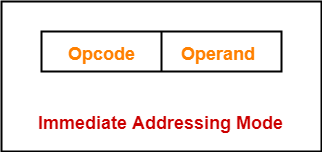## Examples-

• ADD 10 will increment the value stored in the accumulator by 10.
• MOV R #20 initializes register R to a constant value 20.

• The address field of the instruction contains the effective address of the operand.
• Only one reference to memory is required to fetch the operand.
• It is also called as absolute addressing mode.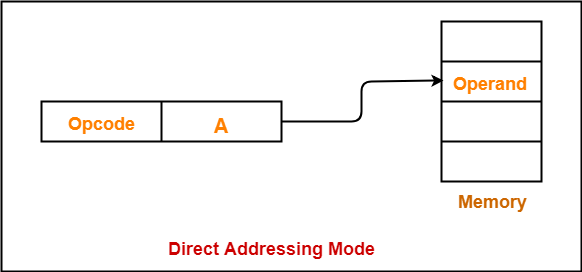## Example-

• ADD X will increment the value stored in the accumulator by the value stored at memory location X.

AC ← AC + [X]

• The address field of the instruction specifies the address of memory location that contains the effective address of the operand.
• Two references to memory are required to fetch the operand.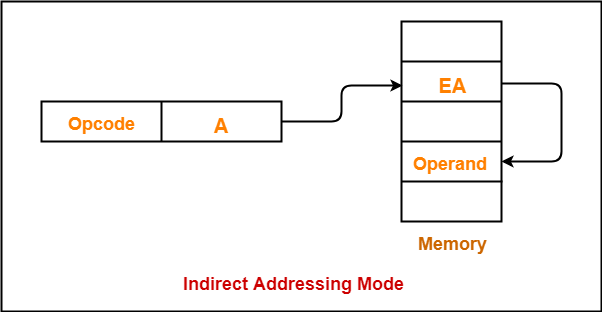## Example-

• ADD X will increment the value stored in the accumulator by the value stored at memory location specified by X.

AC ← AC + [[X]]

## 6. Register Direct Addressing Mode-

• The operand is contained in a register set.
• The address field of the instruction refers to a CPU register that contains the operand.
• No reference to memory is required to fetch the operand.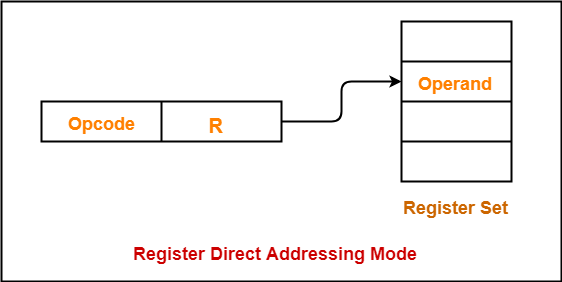## Example-

• ADD R will increment the value stored in the accumulator by the content of register R.

AC ← AC + [R]

## NOTE-

It is interesting to note-

• The only difference is address field of the instruction refers to a CPU register instead of main memory.

## 7. Register Indirect Addressing Mode-

• The address field of the instruction refers to a CPU register that contains the effective address of the operand.
• Only one reference to memory is required to fetch the operand.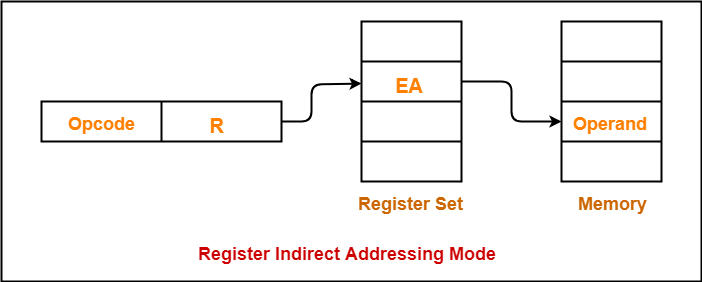## Example-

• ADD R will increment the value stored in the accumulator by the content of memory location specified in register R.

AC ← AC + [[R]]

## NOTE-

It is interesting to note-

• The only difference is address field of the instruction refers to a CPU register.

• Effective address of the operand is obtained by adding the content of program counter with the address part of the instruction.

 Effective Address= Content of Program Counter + Address part of the instruction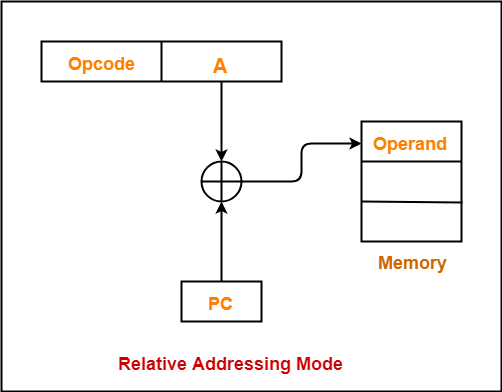## NOTE-

• Program counter (PC) always contains the address of the next instruction to be executed.
• After fetching the address of the instruction, the value of program counter immediately increases.
• The value increases irrespective of whether the fetched instruction has completely executed or not.

• Effective address of the operand is obtained by adding the content of index register with the address part of the instruction.

 Effective Address= Content of Index Register + Address part of the instruction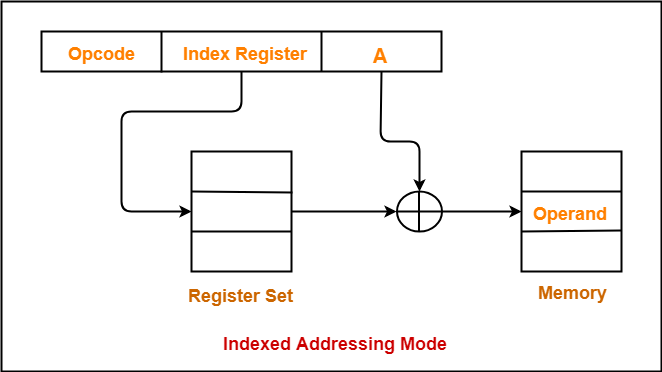## 10. Base Register Addressing Mode-

• Effective address of the operand is obtained by adding the content of base register with the address part of the instruction.

 Effective Address= Content of Base Register + Address part of the instruction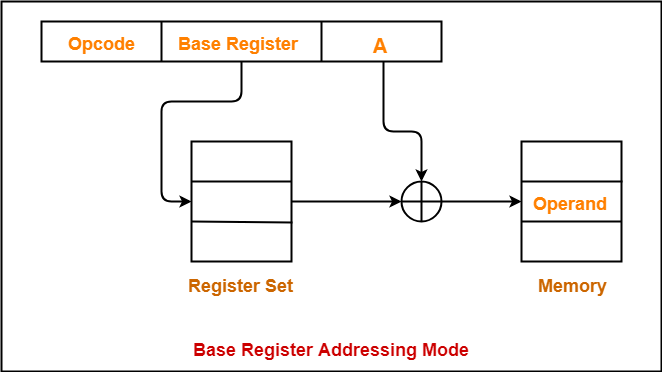• This addressing mode is a special case of Register Indirect Addressing Mode where-

 Effective Address of the Operand= Content of Register

• After accessing the operand, the content of the register is automatically incremented by step size ‘d’.
• Step size ‘d’ depends on the size of operand accessed.
• Only one reference to memory is required to fetch the operand.

## Example-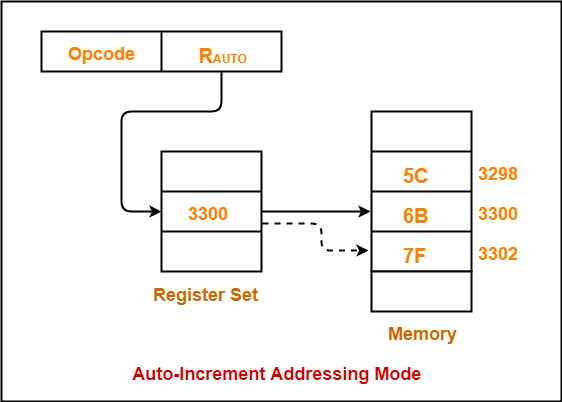Assume operand size = 2 bytes.

Here,

• After fetching the operand 6B, the instruction register RAUTO will be automatically incremented by 2.
• Then, updated value of RAUTO will be 3300 + 2 = 3302.
• At memory address 3302, the next operand will be found.

## NOTE-

• First, the operand value is fetched.
• Then, the instruction register RAUTO value is incremented by step size ‘d’.

• This addressing mode is again a special case of Register Indirect Addressing Mode where-

 Effective Address of the Operand= Content of Register – Step Size

• First, the content of the register is decremented by step size ‘d’.
• Step size ‘d’ depends on the size of operand accessed.
• After decrementing, the operand is read.
• Only one reference to memory is required to fetch the operand.

## Example-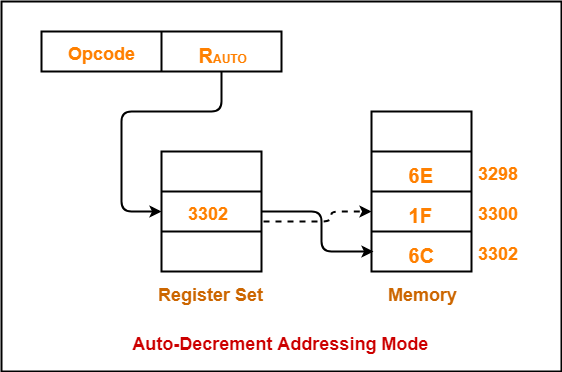Assume operand size = 2 bytes.

Here,

• First, the instruction register RAUTO will be decremented by 2.
• Then, updated value of RAUTO will be 3302 – 2 = 3300.
• At memory address 3300, the operand will be found.

## NOTE-

• First, the instruction register RAUTO value is decremented by step size ‘d’.
• Then, the operand value is fetched.